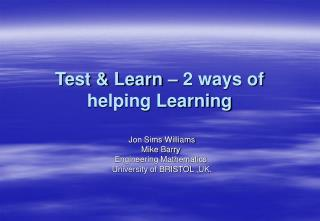DownloadDownload PresentationTest & Learn – 2 ways of helping Learning

# Test & Learn – 2 ways of helping Learning

Download Presentation## Test & Learn – 2 ways of helping Learning

- - - - - - - - - - - - - - - - - - - - - - - - - - - E N D - - - - - - - - - - - - - - - - - - - - - - - - - - -
##### Presentation Transcript

1. Test & Learn – 2 ways of helping Learning Jon Sims Williams Mike Barry Engineering Mathematics University of BRISTOL,UK.

2. A Learning Cycle – Test & Learn - TAL Attend Lecture take test Or Read Book revise Take specialised test

3. TAL – Test & Learn - www.tal.bris.ac.uk • TAL is a Computer Assisted Assessment System • The Core element is an Oracle Database • The database stores questions and student data • about 2000 questions on Mathematics • Both staff and students can talk to TAL from a web browser from home or work

4. Delivery of Support through Tests • Tests provide students with encouragement • Tests tell students where they do not understand • Tests can provide feedback on questions to help students who don’t understand • TAL will build sets of tests that are all on the same topics; take about the same length of time to do and are about of equal difficulty

5. Question :Given x, y, z are real quantities and a, b integer powers, show that the indices in the identity (xayz-1)b(2yz2)2(x/y)a = 4(x6z)2 must be one of the following. Specify which is correct. Answer1:a = 4, b = 2 Feedback: Correct. Answer 2:a = 2, b = 4 Feedback:No. Multiply out and then equate powers of each variable x , y and z in turn Answer 3: a = 3, b = 2 …… TAL Classification: mathematics/Algebra/ Equating powers in identities Facility: 50% Time-to-do: 90 seconds Theory: 3 Function Type: Simple algebraic functions Evaluationtype: Numerical Example Multiple Choice Question

6. Part of Subject Classification • Algebra • Arithmetic • Complex Algebra • Differentiation • Chain; product; ratio rules • Differentiability • Equations of lines • Function of function rule • ….. • Fourier Series • Functions • Etc.

7. TAL and Maths Classification • Weissteins World of Maths – http://mathworld.wolfram.com/ • The European Society for Engineering Education, SEFI syllabus for Core Zero http://learn.lboro.ac.uk/mwg/core.html

8. A small sample from the SEFI syllabus • Analysis and Calculus • Rates of change and differentiation • Average & instantaneous rates of change • Definition of derivative at point • Derivative as instantaneous change rate • Derivative as gradient at a point • Difference between derivative & derived function • Use notations: dy/dx, f(x), y etc.

9. A Theory of Successful Learning successful learning = Ease_of_Learning * Motivation

10. A Learning Cycle – Test & Learn - TAL Attend Lecture take test Or Read Book revise Take specialised test

12. An Example MCQ

13. A Theory of Successful Learning – revisited successful learning = Motivation* Ease_of_Learning

14. Motivation for Histogram

15. The Camera’s Histogram records the number of pixels with each of 255 illumination levels, grouped as shown.

16. How does the camera display the histogram • For each of the 255 illumination levels, cj that the camera can recognise it will do a test to see if the illumination level, I satisfies: cj < I < cj+1 • count the number of pixels that satisfy this constraint. • This then forms the height of the histogram at the point corresponding to cj

17. A better distribution of brightness

18. Pedagogy Support 2 • Example of Motivation but suppose the students did not understand support webpage • So we need to find out why. • Pedagogy Support 2 is a trace of the syllabus back from any point. So we start from Histograms.

19. Trace back through syllabus • Interpret data presented in the form of histograms Requires: • • understand the Cartesian co-ordinate system • • plot points on a graph using Cartesian co-ordinates

20. plot points on a graph using Cartesian co-ordinates requires: • Algebraic expressions and formulae • add and subtract algebraic expressions and simplify the result • understand the terms direct proportion, inverse proportion and joint proportion • solve simple problems involving proportion • interpret simple inequalities in terms of intervals on the real line

21. Summary – 2 ways to support learning • Automatic feedback from the question topic to a website to help the student • Trace back through syllabus to find source of problem • Contact: • Jon.sims.williams@bris.ac.uk to use TAL • Mike.Barry@bris.ac.uk for syllabus tracking